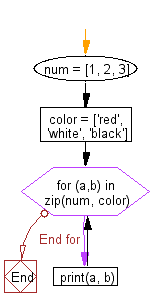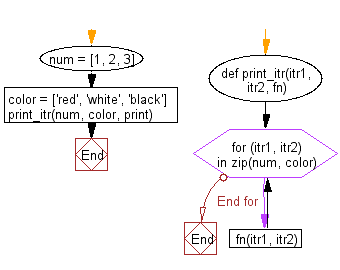﻿ Python: Iterate over two lists simultaneously - w3resource# Python: Iterate over two lists simultaneously

## Python List: Exercise - 64 with Solution

Write a Python program to iterate over two lists simultaneously.

Sample Solution-1:

Python Code:

``````num = [1, 2, 3]
color = ['red', 'white', 'black']
for (a,b) in zip(num, color):
print(a, b)
```
```

Sample Output:

```1 red
2 white
3 black
```

Pictorial Presentation:Flowchart:## Visualize Python code execution:

The following tool visualize what the computer is doing step-by-step as it executes the said program:

Sample Solution-2:

Python Code:

``````def print_itr(itr1, itr2, fn):
for (itr1, itr2) in zip(num, color):
fn(itr1, itr2)
num = [1, 2, 3]
color = ['red', 'white', 'black']
print_itr(num, color, print)
```
```

Sample Output:

```1 red
2 white
3 black
```

Flowchart:## Visualize Python code execution:

The following tool visualize what the computer is doing step-by-step as it executes the said program:

Python Code Editor:

Have another way to solve this solution? Contribute your code (and comments) through Disqus.

What is the difficulty level of this exercise?

Test your Python skills with w3resource's quiz

﻿

## Python: Tips of the Day

Floor Division:

When we speak of division we normally mean (/) float division operator, this will give a precise result in float format with decimals.

For a rounded integer result there is (//) floor division operator in Python. Floor division will only give integer results that are round numbers.

```print(1000 // 300)
print(1000 / 300)```

Output:

```3
3.3333333333333335```